Related Articles

# Doubly Circular Linked List | Set 1 (Introduction and Insertion)

• Difficulty Level : Easy
• Last Updated : 12 Aug, 2021

Circular Doubly Linked List has properties of both doubly linked list and circular linked list in which two consecutive elements are linked or connected by previous and next pointer and the last node points to first node by next pointer and also the first node points to last node by the previous pointer.

Following is representation of a Circular doubly linked list node in C/C++:

```// Structure of the node
struct node
{
int data;
struct node *next; // Pointer to next node
struct node *prev; // Pointer to previous node
};```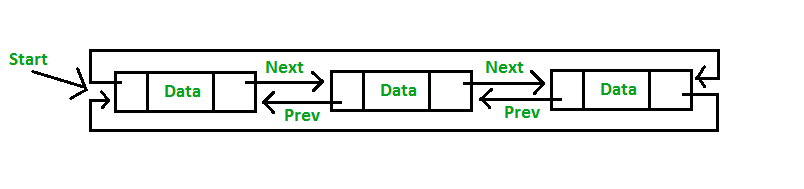Insertion in Circular Doubly Linked List

Insertion in Circular Doubly Linked List

• Insertion at the end of list or in an empty list
• Empty List (start = NULL): A node(Say N) is inserted with data = 5, so previous pointer of N points to N and next pointer of N also points to N. But now start pointer points to the first node the list.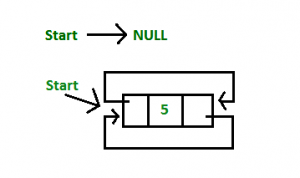• List initially contains some nodes, start points to first node of the List: A node(Say M) is inserted with data = 7, so previous pointer of M points to last node, next pointer of M points to first node and last node’s next pointer points to this M node and first node’s previous pointer points to this M node.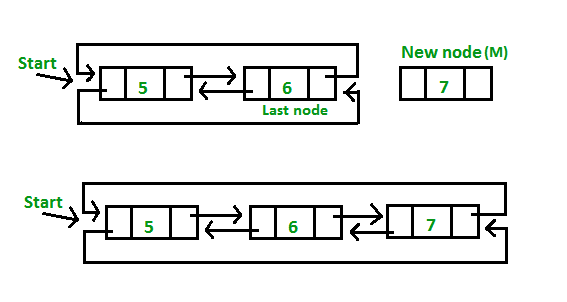## C++

 `// Function to insert at the end``void` `insertEnd(``struct` `Node** start, ``int` `value)``{``    ``// If the list is empty, create a single node``    ``// circular and doubly list``    ``if` `(*start == NULL)``    ``{``        ``struct` `Node* new_node = ``new` `Node;``        ``new_node->data = value;``        ``new_node->next = new_node->prev = new_node;``        ``*start = new_node;``        ``return``;``    ``}` `    ``// If list is not empty` `    ``/* Find last node */``    ``Node *last = (*start)->prev;` `    ``// Create Node dynamically``    ``struct` `Node* new_node = ``new` `Node;``    ``new_node->data = value;` `    ``// Start is going to be next of new_node``    ``new_node->next = *start;` `    ``// Make new node previous of start``    ``(*start)->prev = new_node;` `    ``// Make last previous of new node``    ``new_node->prev = last;` `    ``// Make new node next of old last``    ``last->next = new_node;``}`

## Java

 `// Function to insert at the end``static` `void` `insertEnd(``int` `value)``{``    ` `    ``// If the list is empty, create a single``      ``// node circular and doubly list``    ``if` `(start == ``null``)``    ``{``        ``Node new_node = ``new` `Node();``        ``new_node.data = value;``        ``new_node.next = new_node.prev = new_node;``        ``start = new_node;``        ``return``;``    ``}`` ` `    ``// If list is not empty`` ` `    ``// Find last node``    ``Node last = (start).prev;`` ` `    ``// Create Node dynamically``    ``Node new_node = ``new` `Node();``    ``new_node.data = value;`` ` `    ``// Start is going to be``      ``// next of new_node``    ``new_node.next = start;`` ` `    ``// Make new node previous of start``    ``(start).prev = new_node;`` ` `    ``// Make last previous of new node``    ``new_node.prev = last;`` ` `    ``// Make new node next of old last``    ``last.next = new_node;``}` `// This code is contributed by rutvik_56`

## Python3

 `# Function to insert at the end``def` `insertEnd(value) :``global` `start` `# If the list is empty, create a``# single node circular and doubly list``if` `(start ``=``=` `None``) :` `    ``new_node ``=` `Node(``0``)``    ``new_node.data ``=` `value``    ``new_node.``next` `=` `new_node.prev ``=` `new_node``    ``start ``=` `new_node``    ``return` `# If list is not empty` `# Find last node */``last ``=` `(start).prev` `# Create Node dynamically``new_node ``=` `Node(``0``)``new_node.data ``=` `value` `# Start is going to be next of new_node``new_node.``next` `=` `start` `# Make new node previous of start``(start).prev ``=` `new_node` `# Make last previous of new node``new_node.prev ``=` `last` `# Make new node next of old last``last.``next` `=` `new_node` `# This code is contributed by shivanisinghss2110`

## C#

 `// Function to insert at the end``static` `void` `insertEnd(``int` `value)``{``    ``Node new_node;``     ` `    ``// If the list is empty, create a single node``    ``// circular and doubly list``    ``if` `(start == ``null``)``    ``{``        ``new_node = ``new` `Node();``        ``new_node.data = value;``        ``new_node.next = new_node.prev = new_node;``        ``start = new_node;``        ``return``;``    ``}`` ` `    ``// If list is not empty`` ` `    ``/* Find last node */``    ``Node last = (start).prev;`` ` `    ``// Create Node dynamically``    ``new_node = ``new` `Node();``    ``new_node.data = value;`` ` `    ``// Start is going to be next of new_node``    ``new_node.next = start;`` ` `    ``// Make new node previous of start``    ``(start).prev = new_node;`` ` `    ``// Make last previous of new node``    ``new_node.prev = last;`` ` `    ``// Make new node next of old last``    ``last.next = new_node;``}` `// This code is contributed by Pratham76`

## Javascript

 ``
• Insertion at the beginning of the list: To insert a node at the beginning of the list, create a node(Say T) with data = 5, T next pointer points to first node of the list, T previous pointer points to last node the list, last node’s next pointer points to this T node, first node’s previous pointer also points this T node and at last don’t forget to shift ‘Start’ pointer to this T node.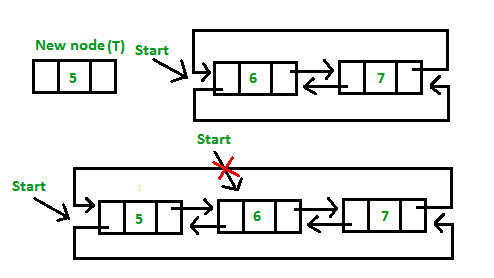## C++

 `// Function to insert Node at the beginning``// of the List,``void` `insertBegin(``struct` `Node** start, ``int` `value)``{``    ``// Pointer points to last Node``    ``struct` `Node *last = (*start)->prev;` `    ``struct` `Node* new_node = ``new` `Node;``    ``new_node->data = value;   ``// Inserting the data` `    ``// setting up previous and next of new node``    ``new_node->next = *start;``    ``new_node->prev = last;` `    ``// Update next and previous pointers of start``    ``// and last.``    ``last->next = (*start)->prev = new_node;` `    ``// Update start pointer``    ``*start = new_node;``}`

## Java

 `// Function to insert Node at the beginning``// of the List,``static` `void` `insertBegin(``int` `value)``{``    ``// Pointer points to last Node``    ``Node last = (start).prev;` `    ``Node new_node = ``new` `Node();``    ``new_node.data = value; ``// Inserting the data` `    ``// setting up previous and next of new node``    ``new_node.next = start;``    ``new_node.prev = last;` `    ``// Update next and previous pointers of start``    ``// and last.``    ``last.next = (start).prev = new_node;` `    ``// Update start pointer``    ``start = new_node;``}` `// this code is contributed by shivanisinghss2110`

## Python3

 `# Function to insert Node at the beginning``# of the List,``def` `insertBegin( value) :``    ``global` `start``    ` `    ``# Pointer points to last Node``    ``last ``=` `(start).prev` `    ``new_node ``=` `Node(``0``)``    ``new_node.data ``=` `value ``# Inserting the data` `    ``# setting up previous and``    ``# next of new node``    ``new_node.``next` `=` `start``    ``new_node.prev ``=` `last` `    ``# Update next and previous pointers``    ``# of start and last.``    ``last.``next` `=` `(start).prev ``=` `new_node``    ` `    ``# Update start pointer``    ``start ``=` `new_node``    ` `    ``# This code is contributed by shivanisinghss2110`

## C#

 `// Function to insert Node at the beginning``// of the List,``static` `void` `insertBegin(``int` `value)``{``  ` `    ``// Pointer points to last Node``    ``Node last = (start).prev;` `    ``Node new_node = ``new` `Node();``    ``new_node.data = value; ``// Inserting the data` `    ``// setting up previous and next of new node``    ``new_node.next = start;``    ``new_node.prev = last;` `    ``// Update next and previous pointers of start``    ``// and last.``    ``last.next = (start).prev = new_node;` `    ``// Update start pointer``    ``start = new_node;``}` `// This code is contributed by shivanisinghss2110`

## Javascript

 `// Function to insert Node at the beginning``      ``// of the List,``      ``function` `insertBegin(value) {``        ``// Pointer points to last Node``        ``var` `last = start.prev;` `        ``var` `new_node = ``new` `Node();``        ``new_node.data = value; ``// Inserting the data` `        ``// setting up previous and next of new node``        ``new_node.next = start;``        ``new_node.prev = last;` `        ``// Update next and previous pointers of start``        ``// and last.``        ``last.next = start.prev = new_node;` `        ``// Update start pointer``        ``start = new_node;``      ``}``      ` `      ``// This code is contributed by shivanisinghss2110`
• Insertion in between the nodes of the list: To insert a node in between the list, two data values are required one after which new node will be inserted and another is the data of the new node.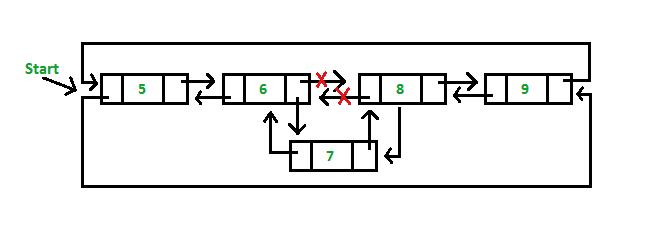## C++

 `// Function to insert node with value as value1.``// The new node is inserted after the node with``// with value2``void` `insertAfter(``struct` `Node** start, ``int` `value1,``                                      ``int` `value2)``{``    ``struct` `Node* new_node = ``new` `Node;``    ``new_node->data = value1; ``// Inserting the data` `    ``// Find node having value2 and next node of it``    ``struct` `Node *temp = *start;``    ``while` `(temp->data != value2)``        ``temp = temp->next;``    ``struct` `Node *next = temp->next;` `    ``// insert new_node between temp and next.``    ``temp->next = new_node;``    ``new_node->prev = temp;``    ``new_node->next = next;``    ``next->prev = new_node;``}`

## Java

 `// Function to insert node with value as value1.``// The new node is inserted after the node with``// with value2``static` `void` `insertAfter(``int` `value1,``                                    ``int` `value2)``{``    ``Node new_node = ``new` `Node();``    ``new_node.data = value1; ``// Inserting the data` `    ``// Find node having value2 and next node of it``    ``Node temp = start;``    ``while` `(temp.data != value2)``        ``temp = temp.next;``    ``Node next = temp.next;` `    ``// insert new_node between temp and next.``    ``temp.next = new_node;``    ``new_node.prev = temp;``    ``new_node.next = next;``    ``next.prev = new_node;``}` `// this code is contributed by shivanisinghss2110`

## Python3

 `# Function to insert node with value as value1.``# The new node is inserted after the node with``# with value2``def` `insertAfter(value1, value2) :``    ``global` `start``    ``new_node ``=` `Node(``0``)``    ``new_node.data ``=` `value1 ``# Inserting the data` `    ``# Find node having value2 and``    ``# next node of it``    ``temp ``=` `start``    ``while` `(temp.data !``=` `value2) :``        ``temp ``=` `temp.``next``    ``next` `=` `temp.``next` `    ``# insert new_node between temp and next.``    ``temp.``next` `=` `new_node``    ``new_node.prev ``=` `temp``    ``new_node.``next` `=` `next``    ``next``.prev ``=` `new_node``    ` `# this code is contributed by shivanisinghss2110`

## C#

 `// Function to insert node with value as value1.``// The new node is inserted after the node with``// with value2``static` `void` `insertAfter(``int` `value1, ``int` `value2)``{``    ``Node new_node = ``new` `Node();``    ``new_node.data = value1; ``// Inserting the data` `    ``// Find node having value2 and next node of it``    ``Node temp = start;``    ``while` `(temp.data != value2)``        ``temp = temp.next;``    ``Node next = temp.next;` `    ``// insert new_node between temp and next.``    ``temp.next = new_node;``    ``new_node.prev = temp;``    ``new_node.next = next;``    ``next.prev = new_node;``}` `// this code is contributed by shivanisinghss2110`

## Javascript

 ``

Following is a complete program that uses all of the above methods to create a circular doubly linked list.

## C++

 `// C++ program to illustrate inserting a Node in``// a Circular Doubly Linked list in begging, end``// and middle``#include ``using` `namespace` `std;` `// Structure of a Node``struct` `Node``{``    ``int` `data;``    ``struct` `Node *next;``    ``struct` `Node *prev;``};` `// Function to insert at the end``void` `insertEnd(``struct` `Node** start, ``int` `value)``{``    ``// If the list is empty, create a single node``    ``// circular and doubly list``    ``if` `(*start == NULL)``    ``{``        ``struct` `Node* new_node = ``new` `Node;``        ``new_node->data = value;``        ``new_node->next = new_node->prev = new_node;``        ``*start = new_node;``        ``return``;``    ``}` `    ``// If list is not empty` `    ``/* Find last node */``    ``Node *last = (*start)->prev;` `    ``// Create Node dynamically``    ``struct` `Node* new_node = ``new` `Node;``    ``new_node->data = value;` `    ``// Start is going to be next of new_node``    ``new_node->next = *start;` `    ``// Make new node previous of start``    ``(*start)->prev = new_node;` `    ``// Make last previous of new node``    ``new_node->prev = last;` `    ``// Make new node next of old last``    ``last->next = new_node;``}` `// Function to insert Node at the beginning``// of the List,``void` `insertBegin(``struct` `Node** start, ``int` `value)``{``    ``// Pointer points to last Node``    ``struct` `Node *last = (*start)->prev;` `    ``struct` `Node* new_node = ``new` `Node;``    ``new_node->data = value;   ``// Inserting the data` `    ``// setting up previous and next of new node``    ``new_node->next = *start;``    ``new_node->prev = last;` `    ``// Update next and previous pointers of start``    ``// and last.``    ``last->next = (*start)->prev = new_node;` `    ``// Update start pointer``    ``*start = new_node;``}` `// Function to insert node with value as value1.``// The new node is inserted after the node with``// with value2``void` `insertAfter(``struct` `Node** start, ``int` `value1,``                                      ``int` `value2)``{``    ``struct` `Node* new_node = ``new` `Node;``    ``new_node->data = value1; ``// Inserting the data` `    ``// Find node having value2 and next node of it``    ``struct` `Node *temp = *start;``    ``while` `(temp->data != value2)``        ``temp = temp->next;``    ``struct` `Node *next = temp->next;` `    ``// insert new_node between temp and next.``    ``temp->next = new_node;``    ``new_node->prev = temp;``    ``new_node->next = next;``    ``next->prev = new_node;``}`  `void` `display(``struct` `Node* start)``{``    ``struct` `Node *temp = start;` `    ``printf``(``"\nTraversal in forward direction \n"``);``    ``while` `(temp->next != start)``    ``{``        ``printf``(``"%d "``, temp->data);``        ``temp = temp->next;``    ``}``    ``printf``(``"%d "``, temp->data);` `    ``printf``(``"\nTraversal in reverse direction \n"``);``    ``Node *last = start->prev;``    ``temp = last;``    ``while` `(temp->prev != last)``    ``{``        ``printf``(``"%d "``, temp->data);``        ``temp = temp->prev;``    ``}``    ``printf``(``"%d "``, temp->data);``}` `/* Driver program to test above functions*/``int` `main()``{``    ``/* Start with the empty list */``    ``struct` `Node* start = NULL;` `    ``// Insert 5. So linked list becomes 5->NULL``    ``insertEnd(&start, 5);` `    ``// Insert 4 at the beginning. So linked``    ``// list becomes 4->5``    ``insertBegin(&start, 4);` `    ``// Insert 7 at the end. So linked list``    ``// becomes 4->5->7``    ``insertEnd(&start, 7);` `    ``// Insert 8 at the end. So linked list``    ``// becomes 4->5->7->8``    ``insertEnd(&start, 8);` `    ``// Insert 6, after 5. So linked list``    ``// becomes 4->5->6->7->8``    ``insertAfter(&start, 6, 5);` `    ``printf``(``"Created circular doubly linked list is: "``);``    ``display(start);` `    ``return` `0;``}`

## Java

 `// Java program to illustrate inserting a Node in``// a Circular Doubly Linked list in begging, end``// and middle``import` `java.util.*;` `class` `GFG``{` `static` `Node start;` `// Structure of a Node``static` `class` `Node``{``    ``int` `data;``    ``Node next;``    ``Node prev;``};` `// Function to insert at the end``static` `void` `insertEnd(``int` `value)``{``    ``// If the list is empty, create a single node``    ``// circular and doubly list``    ``if` `(start == ``null``)``    ``{``        ``Node new_node = ``new` `Node();``        ``new_node.data = value;``        ``new_node.next = new_node.prev = new_node;``        ``start = new_node;``        ``return``;``    ``}` `    ``// If list is not empty` `    ``/* Find last node */``    ``Node last = (start).prev;` `    ``// Create Node dynamically``    ``Node new_node = ``new` `Node();``    ``new_node.data = value;` `    ``// Start is going to be next of new_node``    ``new_node.next = start;` `    ``// Make new node previous of start``    ``(start).prev = new_node;` `    ``// Make last previous of new node``    ``new_node.prev = last;` `    ``// Make new node next of old last``    ``last.next = new_node;``}` `// Function to insert Node at the beginning``// of the List,``static` `void` `insertBegin(``int` `value)``{``    ``// Pointer points to last Node``    ``Node last = (start).prev;` `    ``Node new_node = ``new` `Node();``    ``new_node.data = value; ``// Inserting the data` `    ``// setting up previous and next of new node``    ``new_node.next = start;``    ``new_node.prev = last;` `    ``// Update next and previous pointers of start``    ``// and last.``    ``last.next = (start).prev = new_node;` `    ``// Update start pointer``    ``start = new_node;``}` `// Function to insert node with value as value1.``// The new node is inserted after the node with``// with value2``static` `void` `insertAfter(``int` `value1,``                                    ``int` `value2)``{``    ``Node new_node = ``new` `Node();``    ``new_node.data = value1; ``// Inserting the data` `    ``// Find node having value2 and next node of it``    ``Node temp = start;``    ``while` `(temp.data != value2)``        ``temp = temp.next;``    ``Node next = temp.next;` `    ``// insert new_node between temp and next.``    ``temp.next = new_node;``    ``new_node.prev = temp;``    ``new_node.next = next;``    ``next.prev = new_node;``}` `static` `void` `display()``{``    ``Node temp = start;` `    ``System.out.printf(``"\nTraversal in forward direction \n"``);``    ``while` `(temp.next != start)``    ``{``        ``System.out.printf(``"%d "``, temp.data);``        ``temp = temp.next;``    ``}``    ``System.out.printf(``"%d "``, temp.data);` `    ``System.out.printf(``"\nTraversal in reverse direction \n"``);``    ``Node last = start.prev;``    ``temp = last;``    ``while` `(temp.prev != last)``    ``{``        ``System.out.printf(``"%d "``, temp.data);``        ``temp = temp.prev;``    ``}``    ``System.out.printf(``"%d "``, temp.data);``}` `/* Driver code*/``public` `static` `void` `main(String[] args)``{``    ``/* Start with the empty list */``    ``Node start = ``null``;` `    ``// Insert 5. So linked list becomes 5.null``    ``insertEnd(``5``);` `    ``// Insert 4 at the beginning. So linked``    ``// list becomes 4.5``    ``insertBegin(``4``);` `    ``// Insert 7 at the end. So linked list``    ``// becomes 4.5.7``    ``insertEnd(``7``);` `    ``// Insert 8 at the end. So linked list``    ``// becomes 4.5.7.8``    ``insertEnd(``8``);` `    ``// Insert 6, after 5. So linked list``    ``// becomes 4.5.6.7.8``    ``insertAfter(``6``, ``5``);` `    ``System.out.printf(``"Created circular doubly linked list is: "``);``    ``display();``}``}` `// This code is contributed by Rajput-Ji`

## Python3

 `# Python3 program to illustrate inserting``# a Node in a Circular Doubly Linked list``# in begging, end and middle``start ``=` `None` `# Structure of a Node``class` `Node:``    ``def` `__init__(``self``, data):``        ``self``.data ``=` `data``        ``self``.``next` `=` `None``        ``self``.prev ``=` `None` `# Function to insert at the end``def` `insertEnd(value) :``    ``global` `start``    ` `    ``# If the list is empty, create a``    ``# single node circular and doubly list``    ``if` `(start ``=``=` `None``) :` `        ``new_node ``=` `Node(``0``)``        ``new_node.data ``=` `value``        ``new_node.``next` `=` `new_node.prev ``=` `new_node``        ``start ``=` `new_node``        ``return` `    ``# If list is not empty` `    ``# Find last node */``    ``last ``=` `(start).prev` `    ``# Create Node dynamically``    ``new_node ``=` `Node(``0``)``    ``new_node.data ``=` `value` `    ``# Start is going to be next of new_node``    ``new_node.``next` `=` `start` `    ``# Make new node previous of start``    ``(start).prev ``=` `new_node` `    ``# Make last previous of new node``    ``new_node.prev ``=` `last` `    ``# Make new node next of old last``    ``last.``next` `=` `new_node` `# Function to insert Node at the beginning``# of the List,``def` `insertBegin( value) :``    ``global` `start``    ` `    ``# Pointer points to last Node``    ``last ``=` `(start).prev` `    ``new_node ``=` `Node(``0``)``    ``new_node.data ``=` `value ``# Inserting the data` `    ``# setting up previous and``    ``# next of new node``    ``new_node.``next` `=` `start``    ``new_node.prev ``=` `last` `    ``# Update next and previous pointers``    ``# of start and last.``    ``last.``next` `=` `(start).prev ``=` `new_node` `    ``# Update start pointer``    ``start ``=` `new_node` `# Function to insert node with value as value1.``# The new node is inserted after the node with``# with value2``def` `insertAfter(value1, value2) :``    ``global` `start``    ``new_node ``=` `Node(``0``)``    ``new_node.data ``=` `value1 ``# Inserting the data` `    ``# Find node having value2 and``    ``# next node of it``    ``temp ``=` `start``    ``while` `(temp.data !``=` `value2) :``        ``temp ``=` `temp.``next``    ``next` `=` `temp.``next` `    ``# insert new_node between temp and next.``    ``temp.``next` `=` `new_node``    ``new_node.prev ``=` `temp``    ``new_node.``next` `=` `next``    ``next``.prev ``=` `new_node` `def` `display() :``    ``global` `start``    ``temp ``=` `start` `    ``print` `(``"Traversal in forward direction:"``)``    ``while` `(temp.``next` `!``=` `start) :` `        ``print` `(temp.data, end ``=` `" "``)``        ``temp ``=` `temp.``next``    ` `    ``print` `(temp.data)``    ` `    ``print` `(``"Traversal in reverse direction:"``)``    ``last ``=` `start.prev``    ``temp ``=` `last``    ``while` `(temp.prev !``=` `last) :` `        ``print` `(temp.data, end ``=` `" "``)``        ``temp ``=` `temp.prev``    ` `    ``print` `(temp.data)` `# Driver Code``if` `__name__ ``=``=` `'__main__'``:``    ``global` `start``    ` `    ``# Start with the empty list``    ``start ``=` `None``    ` `    ``# Insert 5. So linked list becomes 5.None``    ``insertEnd(``5``)` `    ``# Insert 4 at the beginning. So linked``    ``# list becomes 4.5``    ``insertBegin(``4``)` `    ``# Insert 7 at the end. So linked list``    ``# becomes 4.5.7``    ``insertEnd(``7``)` `    ``# Insert 8 at the end. So linked list``    ``# becomes 4.5.7.8``    ``insertEnd(``8``)` `    ``# Insert 6, after 5. So linked list``    ``# becomes 4.5.6.7.8``    ``insertAfter(``6``, ``5``)` `    ``print` `(``"Created circular doubly linked list is: "``)``    ``display()` `# This code is contributed by Arnab kundu`

## C#

 `// C# program to illustrate inserting a Node in``// a Circular Doubly Linked list in begging, end``// and middle``using` `System;``using` `System.Collections.Generic;` `class` `GFG``{``static` `Node start;` `// Structure of a Node``public` `class` `Node``{``    ``public` `int` `data;``    ``public` `Node next;``    ``public` `Node prev;``};` `// Function to insert at the end``static` `void` `insertEnd(``int` `value)``{``    ``Node new_node;``    ` `    ``// If the list is empty, create a single node``    ``// circular and doubly list``    ``if` `(start == ``null``)``    ``{``        ``new_node = ``new` `Node();``        ``new_node.data = value;``        ``new_node.next = new_node.prev = new_node;``        ``start = new_node;``        ``return``;``    ``}` `    ``// If list is not empty` `    ``/* Find last node */``    ``Node last = (start).prev;` `    ``// Create Node dynamically``    ``new_node = ``new` `Node();``    ``new_node.data = value;` `    ``// Start is going to be next of new_node``    ``new_node.next = start;` `    ``// Make new node previous of start``    ``(start).prev = new_node;` `    ``// Make last previous of new node``    ``new_node.prev = last;` `    ``// Make new node next of old last``    ``last.next = new_node;``}` `// Function to insert Node at the beginning``// of the List,``static` `void` `insertBegin(``int` `value)``{``    ``// Pointer points to last Node``    ``Node last = (start).prev;` `    ``Node new_node = ``new` `Node();``    ``new_node.data = value; ``// Inserting the data` `    ``// setting up previous and next of new node``    ``new_node.next = start;``    ``new_node.prev = last;` `    ``// Update next and previous pointers of start``    ``// and last.``    ``last.next = (start).prev = new_node;` `    ``// Update start pointer``    ``start = new_node;``}` `// Function to insert node with value as value1.``// The new node is inserted after the node with``// with value2``static` `void` `insertAfter(``int` `value1, ``int` `value2)``{``    ``Node new_node = ``new` `Node();``    ``new_node.data = value1; ``// Inserting the data` `    ``// Find node having value2 and next node of it``    ``Node temp = start;``    ``while` `(temp.data != value2)``        ``temp = temp.next;``    ``Node next = temp.next;` `    ``// insert new_node between temp and next.``    ``temp.next = new_node;``    ``new_node.prev = temp;``    ``new_node.next = next;``    ``next.prev = new_node;``}` `static` `void` `display()``{``    ``Node temp = start;` `    ``Console.Write(``"\nTraversal in forward direction \n"``);``    ``while` `(temp.next != start)``    ``{``        ``Console.Write(``"{0} "``, temp.data);``        ``temp = temp.next;``    ``}``    ``Console.Write(``"{0} "``, temp.data);` `    ``Console.Write(``"\nTraversal in reverse direction \n"``);``    ``Node last = start.prev;``    ``temp = last;``    ``while` `(temp.prev != last)``    ``{``        ``Console.Write(``"{0} "``, temp.data);``        ``temp = temp.prev;``    ``}``    ``Console.Write(``"{0} "``, temp.data);``}` `/* Driver code*/``public` `static` `void` `Main(String[] args)``{``    ``/* Start with the empty list */``    ``Node start = ``null``;` `    ``// Insert 5. So linked list becomes 5.null``    ``insertEnd(5);` `    ``// Insert 4 at the beginning. So linked``    ``// list becomes 4.5``    ``insertBegin(4);` `    ``// Insert 7 at the end. So linked list``    ``// becomes 4.5.7``    ``insertEnd(7);` `    ``// Insert 8 at the end. So linked list``    ``// becomes 4.5.7.8``    ``insertEnd(8);` `    ``// Insert 6, after 5. So linked list``    ``// becomes 4.5.6.7.8``    ``insertAfter(6, 5);` `    ``Console.Write(``"Created circular doubly linked list is: "``);``    ``display();``}``}` `// This code is contributed by Rajput-Ji`

## Javascript

 ``

Output:

```Created circular doubly linked list is:
Traversal in forward direction
4 5 6 7 8
Traversal in reverse direction
8 7 6 5 4```

• List can be traversed from both directions i.e. from head to tail or from tail to head.
• Jumping from head to tail or from tail to head is done in constant time O(1).
• Circular Doubly Linked Lists are used for the implementation of advanced data structures like Fibonacci Heap.

• It takes slightly extra memory in each node to accommodate the previous pointer.
• Lots of pointers involved while implementing or doing operations on a list. So, pointers should be handled carefully otherwise data of the list may get lost.

Applications of Circular doubly linked list

• Managing songs playlist in media player applications.
• Managing shopping cart in online shopping.

This article is contributed by Akash Gupta. If you like GeeksforGeeks and would like to contribute, you can also write an article using write.geeksforgeeks.org or mail your article to review-team@geeksforgeeks.org. See your article appearing on the GeeksforGeeks main page and help other Geeks.